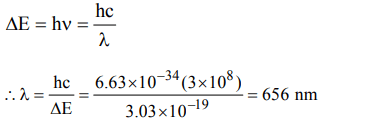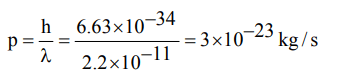## Atomic Structure Questions and Answers Part-5

1. The number of electrons and neutrons of an element is 18 and 20 respectively. Its mass number is
a) 2
b) 17
c) 37
d) 38

Explanation: For neutral atom . No. of p = No. of e- = 18 and A = Z + No. of neutrons = 18 + 20 = 38

2. The hydride ion is isoelectronic with
a) $H^{+}$
b) $He^{+}$
c) He
d) Be

Explanation: Hydride ion H- contains two electrons and He contains two electrons.

3. Which of the following isoelectronic species has the smallest atomic radius?
a) $N^{3-}$
b) $O^{2-}$
c) $F^{-}$
d) Ne

Explanation: $F^{-}$

4. Among the following groupings which represents the collection of isoelectronic species?
a) $NO^{+},C_2^{2-},O_2^-,CO$
b) $N_{2},C_2^{2-},CO,NO$
c) $CO,NO^{+},CN^{-},C_2^{2-}$
d) $NO,CN^{-},N_{2},O_2^-$

Explanation: The species $CO,NO^{+},CN^{-},C_2^{2-}$   Contain 14 electrons each.

5. Which of the following is not iso electronic
a) $Na^{+}$
b) $Mg^{2+}$
c) $O^{2-}$
d) $Cl^{-}$

Explanation: Na+ , Mg++, O2- contain 10 electrons each Cl- has 18 electrons.

6. Chloride ion and potassium ion are isoelectronic. Then
a) their sizes are same
b) chloride ion is bigger than potassium ion
c) potassium ion is relatively bigger
d) depends upon the other cation or anion

Explanation: Nuclear charge of K+ (19) is more than nuclear charge of Cl (17).

7. Ratio of energy of photon of wavelength 3000 Å and 6000Å is
a) 3 : 1
b) 2 : 1
c) 1 : 2
d) 1 : 3

Explanation:8. The energy of a photon is given as $\triangle E\diagup atom = 3.03*10^{-19} J atom^{-1}$     . Then the wavelength $\left(\lambda\right)$ of the photon is.
a) 65.6 nm
b) 656 nm
c) 0.656 nm
d) 6.56 nm

Explanation:9. If wavelength of photon is $2.2×10^{-11}m, h=6.6 ×10^{-34}Js$       , then momentum of photon is
a) $3 × 10^{-23}Kg/s$
b) $3.33× 10^{22}Kg/s$
c) $1.452× 10^{-44}Kg/s$
d) $6.89× 10^{43}Kg/s$